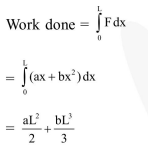# When a rubber-band

Question:

When a rubber-band is stretched by a distance $x$, it exerts a restoring force of magnitude $\mathrm{F}=\mathrm{ax}+\mathrm{bx}^{2}$ where $\mathrm{a}$ and $\mathrm{b}$ are constants. The work done in stretching the unstretched rubberband by L is:-

1. $\frac{a L^{2}}{2}+\frac{b L^{3}}{3}$

2. $\frac{1}{2}\left(\frac{\mathrm{aL}^{2}}{2}+\frac{\mathrm{bL}^{3}}{3}\right)$

3. $a L^{2}+b^{3}$

4. $\frac{1}{2}\left(a L^{2}+b L^{3}\right)$

Correct Option: 1

Solution: